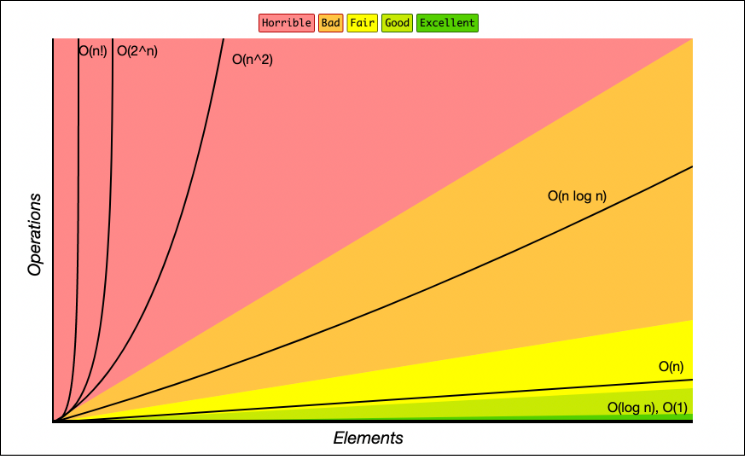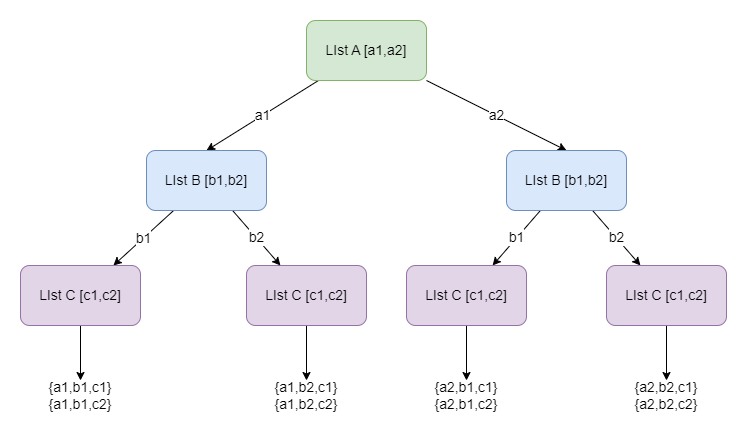• 累计撰写 8 篇文章
• 累计创建 13 个标签
• 累计收到 0 条评论

### 目 录CONTENT# 慢接口优化之回溯算法应用2023-06-03 / 0 评论 / 0 点赞 / 131 阅读 / 2,332 字 / 正在检测是否收录...

## 接口需求

### 数据库建模

CREATE TABLE data_a
(
id       INT AUTO_INCREMENT PRIMARY KEY,
order_id varchar(26) not null default ''
-- 其他相关字段
);
CREATE TABLE data_b
(
id      INT AUTO_INCREMENT PRIMARY KEY,
setting varchar(10)
-- 其他相关字段
);
CREATE TABLE data_c
(
id            INT AUTO_INCREMENT PRIMARY KEY,
progress_info varchar(10)
-- 其他相关字段
);
CREATE TABLE junction
(
id   INT AUTO_INCREMENT PRIMARY KEY,
a_id INT comment '订单表',
b_id INT comment 'KPI规则表',
c_id INT comment '进度表'
);



## 排查思路

1. Redis缓存是否被击穿或穿透？
2. MySQL数据库索引是否合理且正常？
3. 是否有大事务？
4. 代码时间复杂度太高？
5. 本身设计是否合理？

## 排除流程

### 🦀是否有大事务？

#### 处理

• 小批量多线程处理订单信息（多线程还需要考虑数据丢失怎么处理……）
• 清理代码中的事务逻辑，拆分大事务，前置查询等操作

（这篇文章讲算法优化，所以简述事务优化）

### 🦀代码时间复杂度太高？

public class Polymerization {
private String a;
private String b;
private String c;
// 省略
}


private void demo1() {
List<String> a = new ArrayList<>();
List<String> b = new ArrayList<>();
List<String> c = new ArrayList<>();

List<Polymerization> res = new ArrayList<>();
for (String sa : a) {
for (String sb : b) {
for (String sc : c) {
}
}
}
System.out.println(res);
}## 优化思路

### 可用算法

• 分治处理（Divide and Conquer）
• 深度优先搜索回溯（Depth-First Search Backtracking）

#### 分治处理（Divide and Conquer）：

• 思想：将一个大问题划分为若干个子问题，递归地解决子问题，然后将子问题的解合并得到原问题的解。
• 实现：通常通过递归函数来实现。在每一层递归中，将问题分成两个或多个较小的子问题，然后对每个子问题进行递归调用。最后将子问题的解合并得到原问题的解。
• 时间复杂度：通常情况下，分治算法的时间复杂度可以用递推关系式来描述，如T(n) = a * T(n/b) + f(n)，其中a表示划分的子问题个数，b表示问题的规模缩小的比例，f(n)表示合并子问题的时间复杂度。时间复杂度可以通过主定理（Master Theorem）进行求解。

#### 深度优先搜索回溯（Depth-First Search Backtracking）：

• 思想：通过递归地尝试每个可能的解决方案来解决问题。当发现当前尝试的解决方案不能达到目标时，回溯到上一个状态，尝试其他的选择。
• 实现：通常使用递归函数实现。递归函数尝试每个可能的选择，并在每个选择上进行递归调用，然后回溯到上一个状态，继续尝试其他的选择。

### 算法选择

❓既然我们找到两种方案可以解决多重循环结构带来的问题，那我们具体选择什么呢？

private static void dfs(List<List<String>> data, int indexData, int indexA, int indexB, int indexC, Polymerization current) {
if (indexData >= data.size()) {
System.out.println(current);
return;
}
if (indexData == 0) {
for (int i = indexA; i < a.size(); i++) {
current.setA(a.get(i));
dfs(data, indexData + 1, i, indexB, indexC, current);
}
} else if (indexData == 1) {
for (int i = indexB; i < b.size(); i++) {
current.setB(b.get(i));
dfs(data, indexData + 1, indexA, i, indexC, current);
}
} else {
for (int i = indexC; i < c.size(); i++) {
current.setC(c.get(i));
dfs(data, indexData + 1, indexA, indexB, i, current);
}
}
}
private static void demo() {
List<List<String>> data = new ArrayList<>();

dfs(data, 0, 0, 0, 0, new Polymerization());
}


indexData：表示处理对象中的哪个一批属性，业务自己扩展

indexA\indexB\indexC：该指针是为了防止重复匹配到已经用到的属性。可以理解为数据选取的开始位置。

Polymerization{a='a1', b='b1', c='c1'}
Polymerization{a='a1', b='b1', c='c2'}
Polymerization{a='a1', b='b2', c='c1'}
Polymerization{a='a1', b='b2', c='c2'}
Polymerization{a='a2', b='b1', c='c1'}
Polymerization{a='a2', b='b1', c='c2'}
Polymerization{a='a2', b='b2', c='c1'}
Polymerization{a='a2', b='b2', c='c2'}


## 总结：0